# SAS/ETS Examples

## Efficient Method of Moments Estimation of a Stochastic Volatility Model

Contents | SAS Program

# Overview

The Efficient Method of Moments (EMM) is a simulation-based method of estimation that seeks to attain the efficiency of Maximum Likelihood (ML) while maintaining the flexibility of the Generalized Method of Moments (GMM.) This is done using the scores of a pseudo (or auxiliary) model as moment conditions in the GMM step. EMM is particularly useful when the ML approach is not feasible or computationally intensive, such as in models with dynamic latent variables.

In this example, the GMM feature of PROC MODEL is used for implementing the EMM method to estimate a simple stochastic volatility model, where a GARCH(1,1) model serves as the auxiliary model.

# Details

EMM, introduced by Bansal et al. (1993 and 1995) and Gallant and Tauchen (1996), is a variant of the Simulated Method of Moments (SMM) of Duffie and Singleton (1993). The idea is to match the efficiency of the ML estimation with the flexibility of the GMM procedure. ML itself can be interpreted as a method of moment procedure, where the score vector, the vector of derivatives of the log-likelihood function with respect to the parameters, provides the exactly identifying moment conditions. In a first stage, EMM employs an auxiliary (or pseudo) model, which closely matches the true model, for the ML procedure. In a second stage, the scores of the auxilliary model, computed at the ML estimates, provide the moment conditions in the SMM procedure.

EMM is useful for estimating models when the computation of the likelihood function is either infeasible or cumbersome. The typical application involves models with dynamic latent variables, where only one part of the state vector is observable and computation of the likelihood involves integrating out the unobserved part. The dimension of the integral is equivalent to the sample size, so that direct evaluation of the likelihood is not practical. An example of such a model, from Epidemiology, is the SEIR model that describes the parts of a population susceptible, exposed, infected, and recovered from a disease in terms of a system of differential equations, whereas usually the data report only those infected (Olsen and Schaffer 1990). Other examples include stochastic volatility models from Finance, where the instantaneous volatility is unobserved and only the security price can be measured (Gallant and Tauchen 2001), general equilibrium models (Gennote and Marsh 1993), and speculative storage model with rational expectations (Michealides and Ng 2000) from Economics, and compartment models from Pharmacokinetics (Mallet et al. 1988).

Suppose that your data are the time series {y1, y2, ... ,yn }, and the model that you want to estimate, or true model, is characterized by the vector of parameters.

The EMM procedure can be described with the following steps.

1. Projection onto the auxiliary model. Choose an auxiliary model (or score generator) with transition density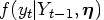parameterized by the pseudo parameter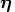, where Yt-1 = {yt-1, ... , y1}. The auxiliary model must approximate the true data generating process as closely as possible and be specified such that ML is feasible.

The only identification requirement is that the dimension of the pseudo parameter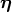be greater or equal to that of the structural parameter.

Estimate then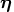with the ML method.

The ML estimator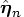satisfies the first order conditions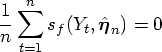where
denotes the score function of the auxiliary model.
2. Covariance matrix computation. Compute a consistent estimator of the asymptotic covariance matrix of the sample pseudo score vector. If the pseudo model is close enough to the structural model, in a suitable sense, Gallant and Long (1997) show that such an estimator may be obtained from the formula3. SMM. For a fixed value of the parameter vector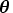, and an arbitrarily large T, simulate the seriesfrom the true model, and find the value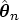that minimizes the quantity
where
is the sample moment condition evaluated at the fixed estimated pseudo parameter. Note that mT depends on the parameteronly through the simulated series.

The Strong Law of Large Numbers guarantees thatconverges almost surely, for, to the population momentwhich, in general, cannot be computed analytically due to the presence of latent variables in the model.

Under suitable conditions, the EMM estimator is consistent and asymptotically normal (Gallant and Tauchen 1996). More precisely, ifand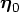are the true values of the parameters of the structural and auxiliary model, respectively, then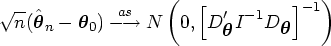where
.

A consistent estimator of the asymptotic variance-covariance matrix of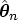is given by
This fact can be used to compute test statistics for the EMM estimator.

# A Stochastic Volatility Model

Consider the following stochastic volatility model for a series of returns yt.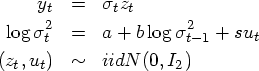for t = 1, ... , n, -1<b<1, s>0, and I2 is the 2-dimensional identity matrix.

The structural parameter setis given by (a, b, s).

The data in this example consist of 1,000 observations generated from the preceding model using the following SAS statements. The values of the parameters are set to (a = -0.736, b = 0.9, s = 0.363).

      /*-------------- Define n and T --------------------*/
%let nobs=1000;
%let nsim=20000;

/* ------------- Generate model data ---------------*/
data _tmpdata;
a = -0.736; b=0.9; s=0.363;
ll=sqrt( exp(a/(1-b)));;
do i=-10 to &nobs;
u = rannor( 101 );
z = rannor( 98761 );
lnssq = a + b*log(ll**2) +s*u;
st = sqrt(exp(lnssq));
ll = st;
y = st * z;
if i > 0 then output;
end;
run;


## Projection onto the GARCH(1,1) Model

Andersen et al. (1999) show that the GARCH(1,1) is an appropriate auxiliary model that leads to a good performance of the EMM estimator for such a structural model.

The analytical expression of the GARCH(1,1) model with mean zero isThe pseudo parameter vectoris given by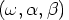.

One advantage of such a class of models is that the conditional density of yt is Gaussian, that is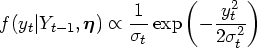and, therefore, the score vector can easily be computed analytically.

The AUTOREG procedure provides ML estimates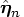. The output is stored in the garchout data set, while the ML estimates are stored in the garchest data set.

      /*-------- estimate GARCH(1,1) model ---------------*/
proc autoreg data=_tmpdata outest=garchest;
model y =  / noint garch=( q=1, p=1 ) ;
output out=garchout cev=gsigmasq r=resid;
run;


## Covariance Matrix Computation

The ML estimates of the GARCH(1,1) model are used in the following SAS DATA step statements to compute the variance-covariance matrix.

      /* --------------- compute the V matrix ------------------*/

data vvalues;
set garchout(keep=y gsigmasq resid);

/* --- compute the scores of the GARCH model -----*/
score_1 = (-1 + y**2/gsigmasq)/ gsigmasq;
score_2 = (-1 + y**2/gsigmasq)*lag(gsigmasq) / gsigmasq;
score_3 = (-1 + y**2/gsigmasq)*lag(y**2) / gsigmasq;

array score{*} score_1-score_3;
array v_t{*} v_t_1-v_t_6;
array v{*} v_1-v_6;

/* - compute the external product of the score vectors - */
do i=1 to 3;
do j=i to 3;
v_t{j*(j-1)/2 + i} = score{i}*score{j};
end;
end;

/* -------- average them over t ------- */
do s=1 to 6;
v{s}+ v_t{s}/&nobs;
end;
run;


The last observation of the vvalues data set contains the values of thematrix.

Obs v_1 v_2 v_3 v_4 v_5 v_6
1000 7315358.77 5282.53 4.37633 2803.39 2.36076 5.53660

Figure 1: Values of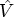Thematrix must be formatted to satisfy the requirements of the VDATA= option of the MODEL procedure. Please refer to the "Input Data Sets" section in "The Model Procedure" chapter of the SAS/ETS User's Guide for more information regarding the VDATA= data set.

      /* --------- Create a dataset acceptable to PROC MODEL --------- */

/* ---- Transpose the last obs in the dataset ----- */
proc transpose data=vvalues(firstobs=&nobs keep=v_1-v_6) out=tempv;
run;

/* ----- Add eq and instrument labels -----*/
data vhat;
set tempv(drop=_name_);
value = col1;
drop col1;
input _type_ $eq_row$ eq_col $inst_row$ inst_col $; datalines; gmm m1 m1 1 1 /* intcpt is the only instrument */ gmm m1 m2 1 1 gmm m2 m2 1 1 gmm m1 m3 1 1 gmm m2 m3 1 1 gmm m3 m3 1 1 ; run;  The vhat data set contains the formatted matrix. Obs value _type_ eq_row eq_col inst_row inst_col 1 7315358.77 gmm m1 m1 1 1 2 5282.53 gmm m1 m2 1 1 3 4.38 gmm m2 m2 1 1 4 2803.39 gmm m1 m3 1 1 5 2.36 gmm m2 m3 1 1 6 5.54 gmm m3 m3 1 1 Figure 2: Formatted## SMM The following statements generate two independent random sequences of length T=20,000 for the errors and utilize them to find the EMM estimates for the structural model using the GMM option of the FIT statement in the MODEL procedure. Please note that the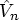matrix serves as weighting matrix in the VDATA= option, and that the scores of the GARCH(1,1) auxiliary model evaluated at the ML estimates are the moment conditions in the GMM step. Since the number of parameters to estimate (3) is equal to the number of moment equations (3) times the number of instruments (1), the model is exactly identified and the objective function will have value zero at the minimum.  /* ---------------------- FIND EMM ESTIMATES ---------------------*/ /* ------- Simulate the error sequences with datastep -------*/ data emm; set garchest(obs=1 keep = _mse_ _ah_0 _ah_1 _gh_1); do i=1 to &nsim; u = rannor( 8801 ); z = rannor( 9701 ); output; end; drop i; run; /* ------------- Estimate parameters with GMM -----------------*/ title 'EMM Estimates'; proc model data=emm; parms a -.7 b .9 s .363; instrument _exog_ / intonly; /*-- Describe the structural model ----*/ lsigmasq = xlag(sigmasq,exp(a)); lnsigmasq = a + b * log(lsigmasq) + s * u; sigmasq = exp( lnsigmasq ); ysim = sqrt(sigmasq) * z; /*--- Volatility of the GARCH model ---*/ gsigmasq = _ah_0 + _gh_1*xlag(gsigmasq, _mse_) + _ah_1*xlag(ysim**2, _mse_); /*---- Scores of the GARCH model as moment conditions ----*/ eq.m1 = (-1 + ysim**2/gsigmasq)/ gsigmasq; eq.m2 = (-1 + ysim**2/gsigmasq)*xlag(gsigmasq, _mse_) / gsigmasq; eq.m3 = (-1 + ysim**2/gsigmasq)*xlag(ysim**2, _mse_) / gsigmasq; /*----- Fit the GARCH scores using GMM and estimated Vhat -----*/ fit m1 m2 m3 / gmm vdata=vhat kernel=(bart,0,) no2sls; bounds s > 0, -1<b<1; run;  The output of the MODEL procedure is as follows:  EMM Estimates The MODEL Procedure Model Summary Parameters 3 Equations 3 Number of Statements 12  Parameters(Value) a(-0.7) b(0.9) s(0.363) m1 m2 m3 The 3 Equations to Estimate m1 = F(a, b, s) m2 = F(a, b, s) m3 = F(a, b, s) Instruments 1  EMM Estimates The MODEL Procedure Nonlinear GMM Parameter Estimates Parameter Estimate Approx Std Err t Value Approx Pr > |t| a -0.50466 0.0778 -6.49 <.0001 b 0.928844 0.0107 87.05 <.0001 s 0.294869 0.0304 9.71 <.0001 Figure 3: PROC MODEL Output ## Computational Considerations The SMM portion of the EMM estimation procedure relies on the simulated series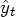. Different random seeds will generate different series, and, therefore, for any finite T, the EMM estimates will vary according to the random seed chosen. However, for T sufficiently large, this variation is neglible when compared to the variation due to the randomness in the sample. For example, using the code provided in Appendix A, you can see that the standard errors of the EMM estimates for T=20,000, computed with a Monte Carlo experiment using 500 different random seeds for the simulated series, are 0.0052 for a, 0.00071 for b, and 0.0023 for s. You can compare these values to the standard errors due to random variations in the sample as computed in Andersen et al. (1999): 0.60 for a, 0.88 for b, and 0.20 for s. As with all nonlinear estimation problems, the computational time required by the EMM estimation can vary a great deal depending on several factors, particularly the choice of the initial values. If the initial values are sufficiently close to the solution, it may take as few as 30 CPU seconds on a Pentium PIII 500Mhz class computer. If the starting values are far from the solution, it may take a several minutes, or not converge at all, depending on the starting values themselves and on the optimization options in PROC MODEL. For computational convenience, the starting values in the above example are the "true" values of the parameters. It is, however, advisable to choose a grid of starting values and repeat the estimation for each set in the grid. Unfortunately, this task is computationally burdensome, and cannot be accomplished using the START= and STARTITER= options of the FIT statement in PROC MODEL, because such options use the 2SLS method and not the moment conditions provided. If you have access to several computers running SAS, you may use the %Distribute macro of Doninger and Tobias (2001) to accomplish such a task in parallel on all the computers and reduce significantly the overall computational time. The code in Appendix A can be easily modified to this purpose. # Appendix A This appendix contains the code for a Monte Carlo study on the properties of the EMM estimator for the stochastic volatility model. The series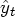is simulated using 500 different seeds for each length T, starting from T=20,000 to T=200,000, at intervals of 20,000, and the EMM estimates are computed for each generated series. The distributions of the resulting estimates are summarized by series length using PROC MEANS. This task is accomplished with parallel computing on seven PCs thanks to the %Distribute macro described in Doninger and Tobias (2001). User names, passwords, and computer addresses have been altered to protect confidentiality.  /***************************************************************/ /* Filename: EMM_DISTR.SAS */ /* Purpose: study distribution of EMM estimates using parallel */ /* distributed computing with %Distribute */ /* Date: 10/03/01 */ /* Modified: */ /* Author: Snow White */ /***************************************************************/ /* / Define libraries where to save results. /------------------------------------------------------------------*/ libname EmmDist "u:\distrib"; /* for pcs */ /* / Include %Distribute and other relevant macros / -----------------------------------------------------------------*/ %inc "u:\distrib\Distribute.sas" / nosource; /* --------------------- UNDISTRIBUTED PART ---------------------- */ /* --------- MACRO for DATA, GARCH ESTIMATES AND VHAT ---------- */ %macro gen_data( nobs = 1000, /* number of obs in the observed data set */ vhat = EmmDist.vhat, /* name of the data set for Vhat */ garchest = EmmDist.garchest, /* data set for GARCH estimates */ seed = 101 /* seed for u and z */ ); data _tmpdata; a = -0.736; b=0.9; s=0.363; ll=sqrt( exp(a/(1-b)));; do i=-10 to &nobs; u = rannor( &seed ); z = rannor( &seed ); lnssq = a + b*log(ll**2) +s*u; st = sqrt(exp(lnssq)); ll = st; y = st * z; if i > 0 then output; end; run; /*-------- estimate GARCH(1,1) model ---------------*/ proc autoreg data=_tmpdata outest=&garchest; model y = / noint garch=( q=1, p=1 ) ; output out=garchout cev=gsigmasq r=resid; run; quit; /* --------------- compute the V matrix ------------------*/ data vvalues; set garchout(keep=y gsigmasq resid); /* --- compute the scores of the GARCH model -----*/ score_1 = (-1 + y**2/gsigmasq)/ gsigmasq; score_2 = (-1 + y**2/gsigmasq)*lag(gsigmasq) / gsigmasq; score_3 = (-1 + y**2/gsigmasq)*lag(y**2) / gsigmasq; array score{*} score_1-score_3; array v_t{*} v_t_1-v_t_6; array v{*} v_1-v_6; /* --- compute the external product of the score vectors -- */ do i=1 to 3; do j=i to 3; v_t{3*(i>1) + 2*(i>2)+j-i+1} = score{i}*score{j}; end; end; /* -------- average them over t ------- */ do s=1 to 6; v{s}+ v_t{s}/&nobs; end; run; quit; /* ---------- Create a data set acceptable to PROC MODEL -------- */ /* ---- Transpose the last obs in the data set ----- */ proc transpose data=vvalues(firstobs=&nobs keep=v_1-v_6) out=tempv; run; quit; /* ----- Add eq and instrument labels -----*/ data labels; _type_ = 'gmm'; inst_row ='1'; inst_col ='1'; do i=1 to 3; do j=i to 3; eq_row=compress("m"||j); eq_col=compress("m"||i); output; end; end; drop i j; run; data &vhat; merge labels tempv(drop=_name_); value=col1; drop col1; run; %mend; /* ------------------- END UNDISTRIBUTED PART ------------------- */ /* / Create pc host data set for pcs /------------------------------------------------------------------*/ data _hosts; script= "c:\Program Files\SAS Institute\sas\connect\saslink\tcpwin.scr"; input host$;
datalines;
Doc
Sleepy
Sneezy
Bashful
Happy
Grumpy
Dopey
;
run;

/*
/ Login to hosts.
/------------------------------------------------------------------*/
%let USER = SnowWhite;
%let PASS = apple;
%SignOn;

/*
/ %RINIT macro
/-------------------------------------------------------------- */
%macro RInit;
rsubmit remote=Host&iHost wait=yes macvar=Status&iHost;

%macro FirstRSub;
*options nonotes;

/* create empty output data sets */
data REmm_est; if (0); run;
data REmm_ods; if (0); run;

/* -------- Rsubmit the MACRO for EMM ESTIMATION ------- */
%macro Emm(
garchest=garchest,  /* GARCH estimates                */
vhat = vhat,        /* data set of Vhat                */
Emm_est = REmm_est, /* output data set of estimates    */
Emm_ods = REmm_ods, /* ODS ParameterEstimates data set */
seed = &rem_Seed,   /* seed for u and z               */
nsim = &rem_Nsim    /* number of simulated obs        */
);

/* -- Simulate error sequences with datastep --*/
data _Emm;
set &garchest(obs=1 keep = _mse_ _ah_0 _ah_1 _gh_1);
do i=1 to &nsim;
u = rannor( &seed );
z = rannor( &seed );
output;
end;
drop i;
run;

/* ------ Estimate parameters with GMM ---------*/
ods output ParameterEstimates=_Emm_ods;
ods output show; /* save the par estimates with ods */

proc model data=_Emm outparms=_Emm_est;
parms a -.7 b .9 s .363;
instrument _exog_ / intonly;

/*-- Describe the structural model ----*/

lsigmasq = xlag(sigmasq,exp(a));

lnsigmasq = a + b * log(lsigmasq) + s * u;

sigmasq = exp( lnsigmasq );

ysim = sqrt(sigmasq) * z;

/*--- Volatility of the GARCH model ---*/
gsigmasq = _ah_0 + _gh_1*xlag(gsigmasq, _mse_) +
_ah_1*xlag(ysim**2, _mse_);

/*- Scores of the GARCH model as moment conditions -*/
eq.m1 = (-1 + ysim**2/gsigmasq)/ gsigmasq;

eq.m2 = (-1 + ysim**2/gsigmasq)*xlag(gsigmasq,_mse_)
/ gsigmasq;

eq.m3 = (-1 + ysim**2/gsigmasq)*xlag(ysim**2,_mse_)
/ gsigmasq;

/*- Fit the GARCH scores using GMM and estimated Vhat -*/
fit m1 m2 m3 / gmm vdata=vhat kernel=(bart,0,);
bounds s > 0, -1<b<1;

run;
ods output close;

/* -- add relevant info in Estimates data set -- */
data &Emm_est;
set &Emm_est _Emm_est;
nsim = &nsim;
seed = &seed;
run;
/* -- add relevant info in ODS output data set -- */
data &Emm_ods;
set &Emm_ods _Emm_ods;
nsim = &nsim;
seed = &seed;
run;

%mend;
%mend;

%macro TaskRSub;
%Emm; /* Run 1 EMM estimation at each remote submission */
%mend;
endrsubmit;
%mend;

/*
/ Loop over different simulated series lengths
/------------------------------------------------------------------*/
%macro Emm_Sim(start=20000,
end=200000,
by=20000
);
data EmmDist.Emm_est; if (0); run; /* create empty data sets */
data EmmDist.Emm_ods; if (0); run; /* for output             */
%do nsim=&start %to &end %by &by;
%Distribute;
%RCollect(REmm_est, REmm_est);
data EmmDist.Emm_est;
set EmmDist.Emm_est REmm_est;
run;
%RCollect(REmm_ods, REmm_ods);
data EmmDist.Emm_ods;
set EmmDist.Emm_ods REmm_ods;
run;
%end;
%mend;

/*
/ Define remote length of simulated serie.
/------------------------------------------------------------------*/
%macro Macros;
%syslput rem_Nsim=&nsim;
%mend;

/*
/ Generate observed data and transfer to remote hosts Work dir
/------------------------------------------------------------------*/
%gen_data;
%macro trandata;
%do i=1 %to 7;
data RWork%left(&i).garchest;
set EmmDist.garchest;
run;
data RWork%left(&i).vhat;
set EmmDist.vhat;
run;
%end;
%mend;
%trandata;

/*
/ Arguments for the distribution.
/------------------------------------------------------------------*/
%let NIter    = 1;
%let NIterAll = 500;
%let Debug    = 0;

/*
/ Run all the stuff and analyze results.
/------------------------------------------------------------------*/
%Emm_Sim(start=20000, end=200000, by=20000);

proc means data=EmmDist.Emm_est n mean stderr lclm uclm;
var a b s;
by nsim;
run;

%SignOff;


# References

Andersen, T.G., Chung, H-J. and Sorensen, B.E. (1999), "Efficient Method of Moments Estimation of a Stochastic Volatility Model: A Monte Carlo Study," Journal of Econometrics, 91, 61-87.

Andersen, T.G. and Sorensen, B.E. (1996), "GMM Estimation of a Stochastic Volatility Model: A Monte Carlo Study," Journal of Business and Economic Statistics, 14, 328-352.

Bansal, R., Gallant, A.R., Hussey, R., Tauchen, G.E. (1993), "Computational Aspects of Nonparametric Simulation Estimation." In Belsey, D.A. (Ed.), Computational Techniques for Econometrics and Economic Analysis. Boston, MA: Kluwer Academic Publishers, 3-22.

Bansal, R., Gallant, A.R., Hussey, R., Tauchen, G.E. (1995), "Nonparametric Estimation of Structural Models for High-Frequency Currency Market Data," Journal of Econometrics, 66, 251-287.

Doninger, C. and Tobias, R. (2001), "The %Distribute System for Large-Scale Parallel Computation in the SAS System." [http://www.sas.com/rnd/app/papers/distConnect.pdf] . Cary, NC: SAS Institute, Inc. Accessed 12 October 2001.

Duffie, D. and Singleton, K.J. (1993), "Simulated Moments Estimation of Markov Models of Asset Prices," Econometrica 61, 929-952.

Gallant, A.R. and Long, J. (1997), "Estimating Stochastic Differential Equations Efficiently by Minimum Chi-squared," Biometrika, 84, 125-141.

Gallant, A.R. and Tauchen, G.E. (1996), "Which Moments to Match?" Econometric Theory, 12, 657-681.

Gallant, A.R. and Tauchen, G.E. (2001), "Efficient Method of Moments," Working Paper. [http://www.econ.duke.edu/ get/wpapers/ee.pdf] , accessed 12 September 2001.

Gennotte, G. and Marsh, T.A. (1993), "Variations in Economic Uncertainty and Risk Premiums on Capital Assets," European Economic Revue, 37, 1021-1041.

Mallet, A., Mentré, F., Steimer, J-L., and Lokiec, F. (1988), "Nonparametric Maximum Likelihood Estimation for Population Pharmacokinetics, with Application to Cyclosporine," Journal of Pharmacokinetics and Biofarmaceutics, 16, 311-327.

Michaelides, A. and Ng, S. (2000), "Estimating the Rational Expectations Model of Speculative Storage: A Monte Carlo Comparison of Three Simulation Estimators," Journal of Econometrics, 96(2), 231-266.

Olsen, L.F. and Schaffer, W.M. (1990), "Chaos versus Noisy Periodicity: Alternative Hypotheses for Childhood Epidemics," Science, 249, 499-504.

SAS Institute, Inc. (1999), SAS/ETS User's Guide, Version 8, Volumes 1 and 2, Cary, NC: SAS Institute, Inc.